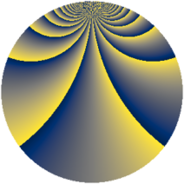# Properties

 Label 2304.3.xLevel $2304$ Weight $3$ Character orbit 2304.x Rep. character $\chi_{2304}(161,\cdot)$ Character field $\Q(\zeta_{8})$ Dimension $256$ Sturm bound $1152$

# Related objects

## Defining parameters

 Level: $$N$$ $$=$$ $$2304 = 2^{8} \cdot 3^{2}$$ Weight: $$k$$ $$=$$ $$3$$ Character orbit: $$[\chi]$$ $$=$$ 2304.x (of order $$8$$ and degree $$4$$) Character conductor: $$\operatorname{cond}(\chi)$$ $$=$$ $$96$$ Character field: $$\Q(\zeta_{8})$$ Sturm bound: $$1152$$

## Dimensions

The following table gives the dimensions of various subspaces of $$M_{3}(2304, [\chi])$$.

Total New Old
Modular forms 3200 256 2944
Cusp forms 2944 256 2688
Eisenstein series 256 0 256

## Trace form

 $$256q + O(q^{10})$$ $$256q + 256q^{61} + O(q^{100})$$

## Decomposition of $$S_{3}^{\mathrm{new}}(2304, [\chi])$$ into newform subspaces

The newforms in this space have not yet been added to the LMFDB.

## Decomposition of $$S_{3}^{\mathrm{old}}(2304, [\chi])$$ into lower level spaces

$$S_{3}^{\mathrm{old}}(2304, [\chi]) \cong$$ $$S_{3}^{\mathrm{new}}(96, [\chi])$$$$^{\oplus 8}$$$$\oplus$$$$S_{3}^{\mathrm{new}}(288, [\chi])$$$$^{\oplus 4}$$$$\oplus$$$$S_{3}^{\mathrm{new}}(384, [\chi])$$$$^{\oplus 4}$$$$\oplus$$$$S_{3}^{\mathrm{new}}(768, [\chi])$$$$^{\oplus 2}$$$$\oplus$$$$S_{3}^{\mathrm{new}}(1152, [\chi])$$$$^{\oplus 2}$$